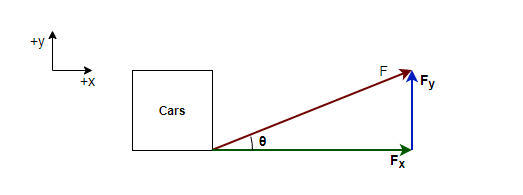# Problem: In April 1974, John Massis of Belgium managed to move two passenger railroad cars. He did so by clamping his teeth down on a bit that was attached to the cars with a rope and then leaning backward while pressing his feet against the railway ties. The cars together weighed 700 kN (about 80 tons). Assume that he pulled with a constant force that was 2.5 times his body weight, at an upward angle θ of 30° from the horizontal. His mass was 80 kg, and he moved the cars by 1.0 m. Neglecting any retarding force from the wheel rotation, find the speed of the cars at the end of the pull.

###### FREE Expert Solution

We're asked for the final velocity of the cars at the end of the pull.

This problem involves forces in kinematics with horizontal acceleration.

Whenever we are given such a problem we apply the following steps.

1. Draw a free body diagram (FBD)
2. Apply kinematics equations and F=ma
3. Solve for the target variable

For step 2, we'll need to use the kinematic equations,

We'll also need, in step 3, equations for getting moments of force:

Step 1: Draw a free body diagram (FBD)

We'll take a coordinate system where the positive x-axis points to the right and positive y-direction points upwardsF is the force applied by John Massis, Fx is the x-component of the force, and Fy is the y-component of the force.

The cars are moving horizontally. So we'll only consider the x-component of force, Fx.

Step 2: Apply kinematics equations and F=ma

89% (98 ratings)###### Problem Details

In April 1974, John Massis of Belgium managed to move two passenger railroad cars. He did so by clamping his teeth down on a bit that was attached to the cars with a rope and then leaning backward while pressing his feet against the railway ties. The cars together weighed 700 kN (about 80 tons). Assume that he pulled with a constant force that was 2.5 times his body weight, at an upward angle θ of 30° from the horizontal. His mass was 80 kg, and he moved the cars by 1.0 m. Neglecting any retarding force from the wheel rotation, find the speed of the cars at the end of the pull.